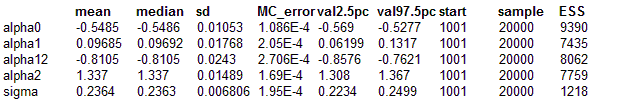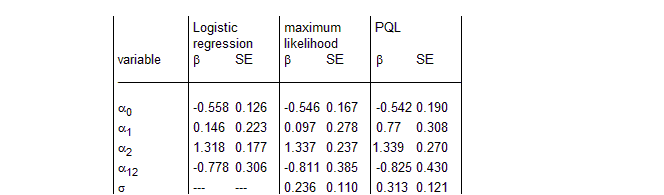#### Using Data Cloning to Calculate MLEs for the Seeds Model

The idea of data cloning allows the user to calculate maximum likelihood estimates via markov chain monte carlo simulation. The procedure works for multilevel models provided the random effects are also replicated. Here we consider the MLE estimates for the seeds model.

Run simulation for K = {1, 2, 4, 8, 16, 32, 64, 128, 256}.. The MLE point estimates are given by the mean of the MCMC simulation and the MLE SE by the MCMC sd scaled by K1/2 .Note that Monte Carlo errors will be magnified by this scaling so accurate estimates of the MLE SE will need both large K and long MCMC runs.

model
{
for( i in 1 : N ) {
for(k in 1 : K){   # replicate data and random effects
r.rep[i, k] <- r[i]
r.rep[i, k] ~ dbin(p[i, k],n[i])

b[i, k] ~ dnorm(0.0,tau)
logit(p[i, k]) <- alpha0 + alpha1 * x1[i] + alpha2 * x2[i] +
alpha12 * x1[i] * x2[i] + b[i, k]
}
}
alpha0 ~ dnorm(0.0,1.0E-6)
alpha1 ~ dnorm(0.0,1.0E-6)
alpha2 ~ dnorm(0.0,1.0E-6)
alpha12 ~ dnorm(0.0,1.0E-6)
tau ~ dgamma(0.001,0.001)
sigma <- 1 / sqrt(tau)
}

Data
##### Inits for chain 1
``` list(alpha0 = 0, alpha1 = 0, alpha2 = 0, alpha12 = 0, sigma = 8) ```

##### Inits for chain 2
``` list(alpha0 = 0, alpha1 = 0, alpha2 = 0, alpha12 = 0, sigma = 1,beta = c(0, 0, 0, 0, 0, 0, 0, 0, 0, 0, 0, 0, 0, 0, 0, 0, 0, 0, 0, 0, 0)) ```

Results

For K = 256We may compare simple logistic, maximum likelihood (from EGRET), penalized quasi-likelihood (PQL) Breslow and Clayton (1993) with the BUGS results using data cloning. Using 256 replicates of the data set we obtain the following MLE
alpha0 = -0.5485 (0.168), alpha1 = 0.09685 (0.283), alpha12 = -0.8105 (0.389),
alpha2 = 1.337 (0.238), sigma = 0.2364 (0.109), in good agreement with the EGRET results.